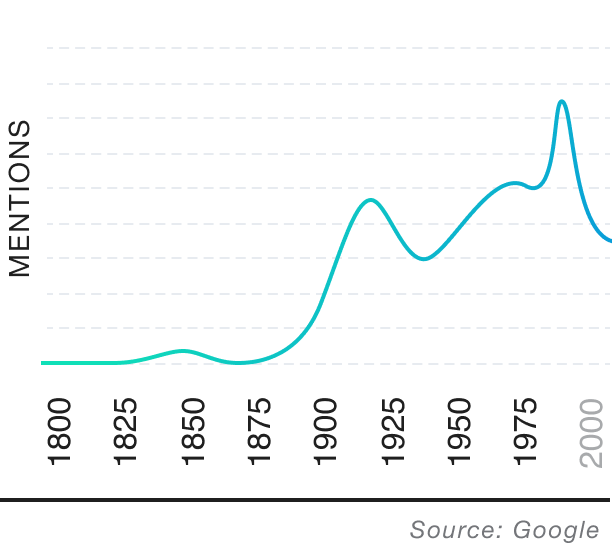All Words > Percentile

[pər-SEN-tiyl]

Part of speech: noun

Origin: English, 19th century

1.

Each of the 100 equal groups into which a population can be divided according to the distribution of values of a particular variable.

2.

Each of the 99 intermediate values of a random variable that divide a frequency distribution into 100 groups.

### Examples ofPercentilein a sentence

"As a baby, Melvin was in above the 95th percentile for height, and he remained taller than his schoolmates all the way to graduation."

"With a score of between 1350 and 1400 on the SAT, a student will rank between the 90th and 94th percentiles, meaning they have scored better than between 90% and 94% of other students."

### Popularity Over Time